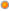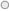# DAX cheat sheet

User Rating: 4 / 5Note: This is work in progress and more samples will be added very soon. Please check back frequently.

Most of the samples are based on "Contoso Samples DAX Formulas.xlsx" available for download here.

### Calculated columns

 DAX Formula Description Additional links =[ChannelLabel] & " - " & [ChannelName] Concatenate string - it is recommended to use operator "&" instead of function "Concatenate" as function accepts just 2 parameters =CONCATENATE( CONCATENATE( [ChannelLabel], " - "), [ChannelName]) Concatenate string =Format([Datekey], "YYYY-MM-DD") Converts date type value to string. Formating options are:YYYY- 4 digit year, example "2010"YY - 2 digit year, example "10"MM - 2 digit month, example "06"MMM - short month name, example "Jun"MMMM - long month name, example "June"DD - 2 digit day, example 07DDD - short weekday name, example "Mon"DDDD - long weekday name, example "Monday" =Date(2010, 6, 1) Create date type field =Date(2010,6,1) + Time(20,15,0) Create date and time type field =Related(DimProductCategory[ProductCategoryName]) Follow relationship and retrieve related value from another table. Example: add this calculation for Product table to calculate related ProductCategory column. =SUMX(RelatedTable(FactSales), FactSales[SalesAmount]) For current row calculate total related sales amountExample: for Product table add column "Total Sales" with this formula that sums sales amounts for ALL product records =COUNTROWS(RelatedTable(FactSales)) For current row calculate related table record countExample: for Product table add column "SalesCount" with this formula that represents total number of sales for this product =AVERAGEX(RelatedTable(FactSales), FactSales[UnitPrice]) For current row calculate average related table column value.Example: for Product table add column "AverageUnitPrice" with this formula that represents average unit price of this product over all sales =SUMX(RELATEDTABLE(FactSales), FactSales[SalesAmount]) / SUM(FactSales[SalesAmount]) For current row calculate percentage of total sales.Example: For ProductCategory table add column "SalesWeight" with this formula that calculates percentage of that ProductCategory sales over grand total sales. This formula sums each Product category total sales and divides by grand total of all sales. =CALCULATE(COUNTROWS(DISTINCT(FactSales[ProductKey]))) For current row calculate number of distinct values from related table.Example: when you add this calculation to dimDate table, then for each row (day) you will get distinct number of ProductKey sold that day.

### Calculated measures

 DAX Formula Description Additional links =COUNTROWS( DISTINCT( FactSales[ProductKey])) Distinct count calculation.Example: number of distinct products sold for current Pivot selection Marco Russo =CALCULATE( SUM(Accounts[Amount]), ( FILTER( VALUES(Accounts[Account]), COUNTROWS(RELATEDTABLE(Cross)) > 0) ) ) Many to many calculation.We have accounts table with amount and customer table. There is also Cross table that contains relationship between customers and accounts. We follow this many-to-many relationship and calculate proper account SUM value Marco Russo = SUMX (FactSales, [UnitPrice] * [SalesQuantity]) Sum of two multiplied columns =SUM(FactSales[SalesAmount]) / CALCULATE(SUM(FactSales[SalesAmount]), ALL(DimProductCategory[ProductCategoryName])) Sales ratio to Product category. We place ProductCategory on row and create calculated measure with this formula. We calculate total sales for this Product category and divide by all ProductCategory total sales.

Time related calculated measures

 Dax Formula Description Additional link Year to Date Last year to date Month to date Last month to date Previous Month =IF( COUNTROWS(VALUES(DimDate[CalendarYear]))=1    , CALCULATE([Sales], PREVIOUSYEAR(DimDate[DateKey]))   , BLANK()) or =IF(  COUNTROWS(VALUES(DimDate[CalendarYear]))=1 ,    CALCULATE([Sales], PARALLELPERIOD(DimDate[Datekey],-12,MONTH))  , BLANK()     ) or =IF(  COUNTROWS(VALUES(DimDate[CalendarYear]))=1 ,   [Sales] (PARALLELPERIOD(DimDate[Datekey],-12,MONTH)),   BLANK()     ) Previous Year =IF(COUNTROWS(VALUES(DimDate[CalendarYear]) = 1  , [Sales] - CALCULATE([Sales], PREVIOUSYEAR(DimDate[Datekey]))  , Blank()) Year over year growth